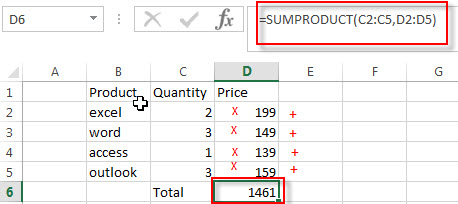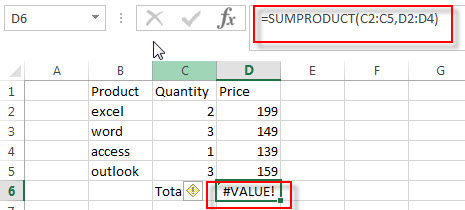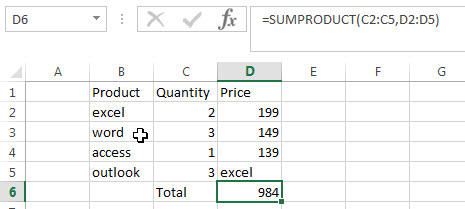# Excel SUMPRODUCT Function

This post will guide you how to use Excel SUMPRODUCT function with syntax and examples in Microsoft excel.

## Description

The Excel SUMPRODUCT function multiplies corresponding components in the given one or more arrays or ranges, and returns the sum of those products. And it returns a numeric value.

The SUMPRODUCT function is a build-in function in Microsoft Excel and it is categorized as a Math and Trigonometry Function.

The SUMPRODUCT function is available in Excel 2016, Excel 2013, Excel 2010, Excel 2007, Excel 2003, Excel XP, Excel 2000, Excel 2011 for Mac.

## Syntax

The syntax of the SUMPRODUCT function is as below:

`= SUMPRODUCT (array1,[array2],…)`

Where the SUMPRODUCT function arguments are:

• array1 -This is a required argument. The first array or range that you want to multiply and then add.
• [array2],…– This is an optional argument.  The second array or range that you want to multiply and then add.

Note:

• The array arguments must have the same dimensions, or it will return the #VALUE! Error.
• The SUMPRODUCT treats non-numeric items in the arrays as zeros.

## Example

The below examples will show you how to use Excel SUMPRODUCT Function to return the sum of products.

#1 To get the total amount of all products in the range B1:B5, just using the following excel formula:

`=SUMPRODUCT(C2:C5,D2:D5)`2# The arrays or ranges must have the same dimensions, otherwise returns the #VALUE error. See the below picture:3# non-numeric items in the arrays or ranges are treated as zeros.### Related Functions

• Excel PRODUCT function
The Excel PRODUCT function multiplies the numbers and returns the product. The syntax of the PRODUCT function is as below:=PRODUCT (number1, [number2], …)…

### More Excel SUMPRODUCT Function Examples

• Count Cells That Contain Specific Text
This post will discuss that how to count the number of cells that contain specific text or certain text in a specified cells of range in Excel. How to get the total number of cells that contain certain text.……
• Count Number of Cells with String Length greater than X
You can create a new formula based on the SUMPRODUCT function, the N function and the LEN function to check the string length of each cell and also get the total number that the string length is greater than number 10.……
• Highlight overlapping dates
If you want to highlight overlapping dates, you need to find the overlapping date ranges firstly, so you need to create a formula based on SUMPRODUCT function, then type this formula into the conditional formatting format box..……
• Find the Largest Value Based on Multiple Criteria
Assuming that you have a list of data that you want to find the largest value based on the product “excel” and the sales region “east”. You can create a new excel formula based on the SUMPRODUCT function and the LARGE function..……
• Sum Cell Value with Case Sensitive
Assuming that you have a list of data in the range A1:B5, and you want to sum cell values based on “excel” product only, and ignore “Excel”. So you can create a new formula based on the SUMPRODUCT function and the EXACT function.…
• Rank Data with Multiple Criteria
Assuming that you want to rank data against multiple criteria for a range of cells in your worksheet. How to achieve it. You need to create a new complex formula based on the SUMPRODUCT function. .…
• Sumif with 3D Reference for Multiple Worksheet
If you want to sum 3d references across the different worksheet, and you need to create a complex formula based on the SUMPRODUCT function, the SUMIF function, and the INDIRECT function..….
• Count Cells between Two Dates with Multiple Criteria
Assuming that you have a list of data that contain two columns,  you want to count the number of product “excel” which is between two dates (from 2018/8/9-2018/10/19) in column B. You can create a new excel formula based on the SUMPRODUCT function to achieve the result.….
• Sum Numbers from 1 to N
Assuming that you supply a number 100, and you want to sum the numbers from 1 to 100 (1+2+3+…+100), you need to create a formula based on the SUMPRODUCT function, the ROW function and the INDIRECT function to achieve the result..….
• Sum if Cells Begin with Specific Text or Number in Another Cells
Assuming that you have a list of data A1:B4, and you want to sum all cells in column B if cells in column A begin with a specific text “excel” or a specific number “201”.You also can create a formula based on the SUMPRODUCT function and the LEFT functio..…
• Count Unique Values Using Pivot Table
You can insert a 3rd or helper column with a formula to check if the value is unique in the selected range of cells, and the create pivot table based on the 1st and 3rd column to count unique values..…
• COUNTIF with Multiple Criteria
To count the number based on multiple criteria in the same range or column in Excel, you can create a formula based on the SUMPRODUCT function and the COUNTIF function.….
• Sum Every Nth Row or Column
If you want to sum every nth rows in Excel, you can create an Excel Array formula based on the SUM function, the MOD function and the ROW function..….
• Count Dates in Given Year/Month/Day in Excel
You can create a formula based on the SUMPRODUCT function and the YEAR function to count dates by a give year….
• Sum the Absolute Values
Assuming that you have a list of data in range B1:B6 that contain negative numbers and positive numbers, and you want to sum all absolute values in these range of cells, You can use a formula based on the SUMIF function to achieve the result….